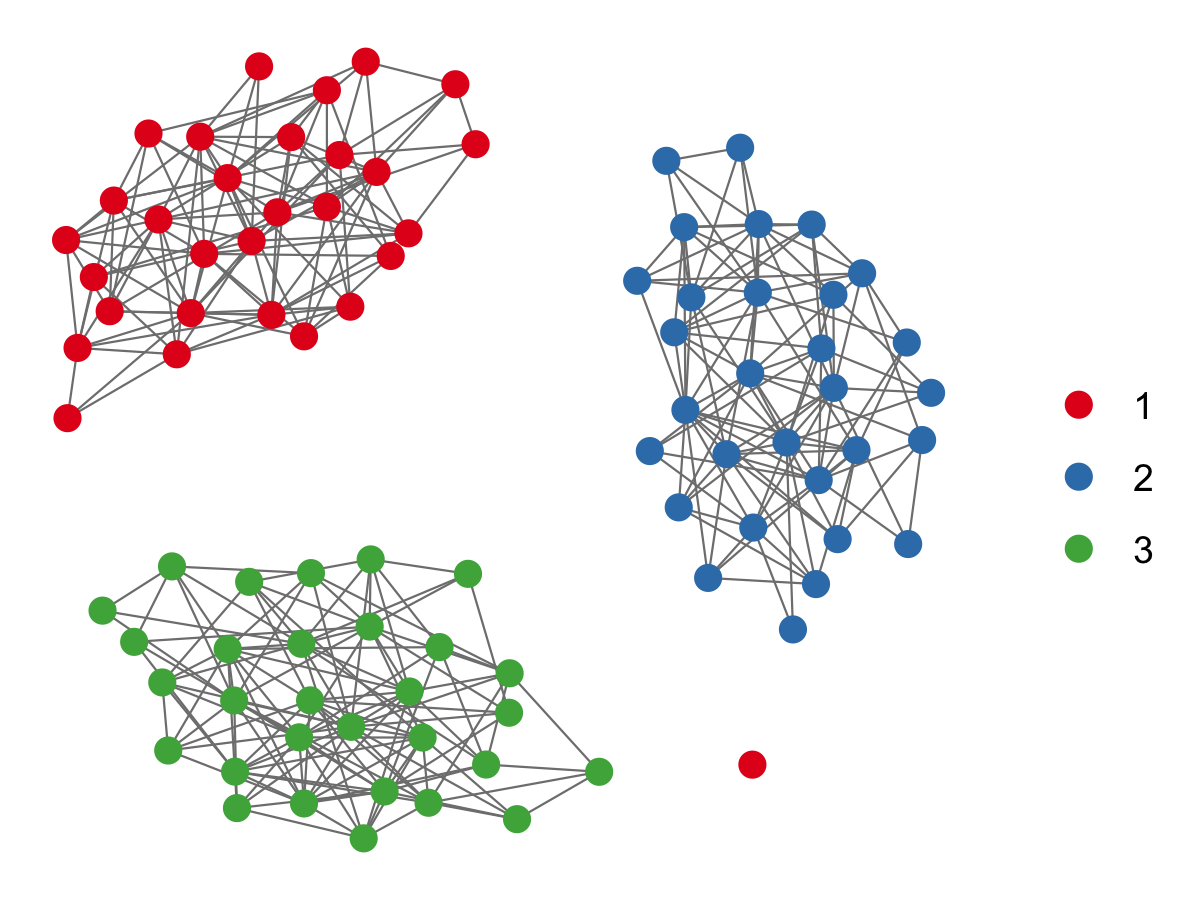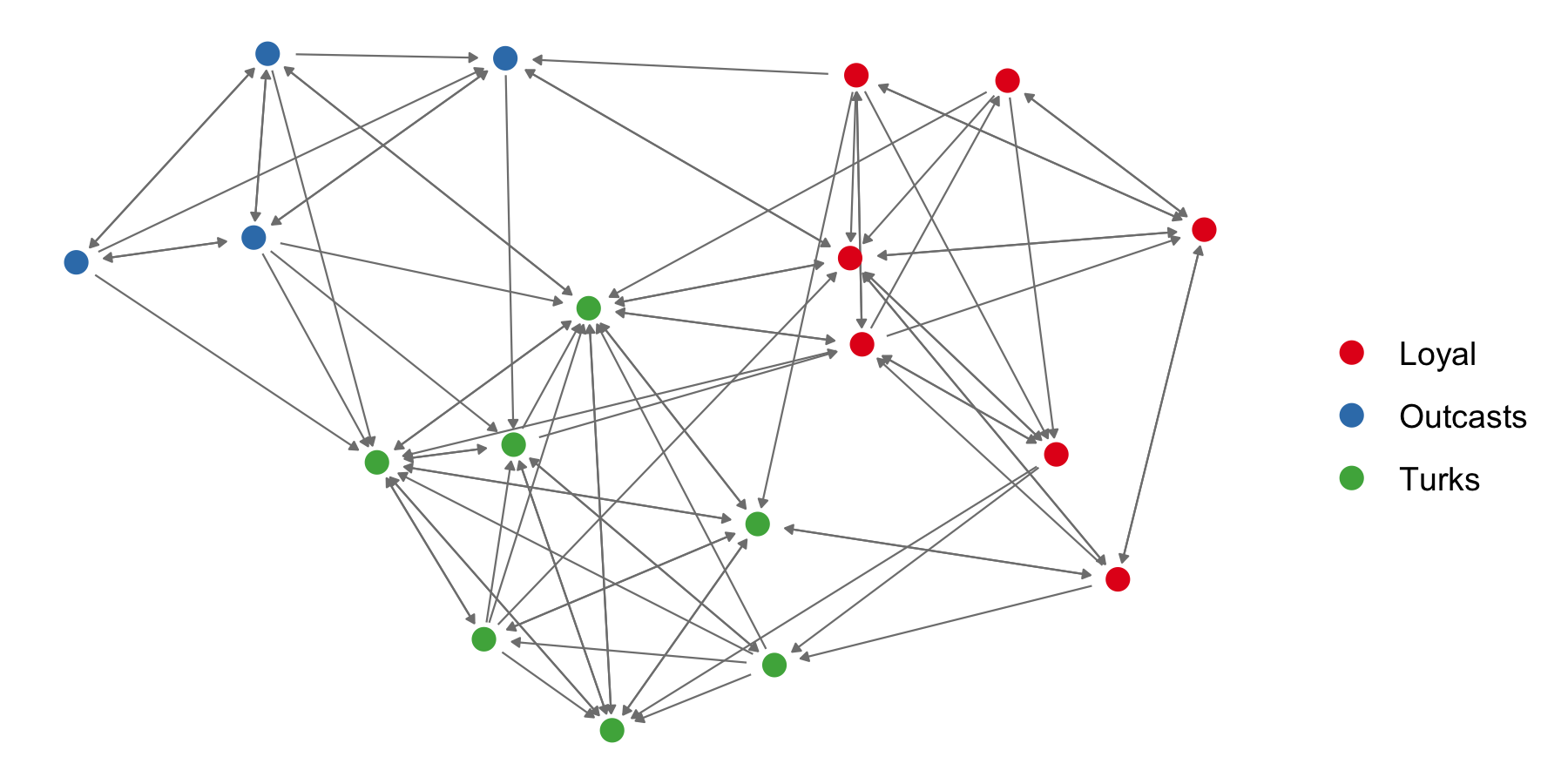## Introduction

The R package mlergm, appropriately named “Multilevel Exponential-Family Random Graph Models,” aims to provide a convenient platform for estimating exponential-family random graph models (ERGMs) with multilevel structure. Presently, the beta release of the package supports estimation of ERGMs with non-overlapping block structure and local dependence (see the work of Schweinberger & Handcock (JRSS-B, 2015)), with plans to expand the coverage to overlapping block structure with local dependence and to structures with multiple levels and higher-order interactions in future updates.

The syntax of mlergm aims to mirror other network package interfaces, namely that of the ergm framework, to provide as small a learning curve as possible to users already acquainted with the ergm and network package framework. In the following sections, we aim to highlight how to use mlergm and some of the key features.

Before we proceed into demonstrating how to use mlergm and the functionality it has for multilevel networks analysis, we first review background on multilevel networks, as well as the main statistical model the package contains at present.

#### Brief background on multilevel networks

Multilevel networks come in many different forms, and we point readers to the introductory chapter written by Snijders in the monograph “Multilevel network analysis for the social sciences: theory, methods and applications” (Lazega & Snijders, Eds., 2016).

In the simplest form, a multilevel network has a set of nodes $$\mathcal{N}$$ (e.g., persons, brain regions, research articles) partitioned into $$K$$ blocks $$\mathcal{A}_{1}, \ldots, \mathcal{A}_{K} \subseteq \mathcal{N}$$ (e.g., departments within a university, individual patient brains, research journals), and a set of edges $$\mathcal{E}$$ which represent interactions, relationships, or connections between nodes (e.g., advice seeking, functional connectivity, citation). Network data are typically represented by an adjacency matrix $$\boldsymbol{X}$$, where in the case of a binary, undirected network, $$X_{i,j} = 1$$ if $$\{i,j\} \in \mathcal{E}$$, and $$X_{i,j} = 0$$ otherwise. The within-block subgraphs are denoted by $$\boldsymbol{X}_{\mathcal{A}_k, \, \mathcal{A}_k}$$ ($$k = 1 , \ldots, K$$) and the between-block subgraphare denoted by $$\boldsymbol{X}_{\mathcal{A}_k, \, \mathcal{A}_l}$$ ($$1 \leq k < l \leq K$$).

In practice, researchers are usually interested in super-population inference, where a network $$X$$, define on a finite population of nodes $$\mathcal{N}$$, is assumed to have been generated from a distribution $$\mathbb{P}_{\mathcal{N}, \, \boldsymbol{\theta}}$$, and the goal is to estimate $$\boldsymbol{\theta}$$ in order to learn about mechanisms driving edge formation.

Past procedures have taken to estimating models for such network data by

1. Estimating an ERGM with no additional structure.
2. Estimating individual ERGMs for each block subgraph.

Both of these approaches fail to take into account the natural structure of networks with block structure. The first is unable to adaptively model differences in within- and between-block edge formations, while the second may overfit to the data by estimating separate parameters for each within-block subgraph, and does not use the additional structure to help estimate a general model.

In mlergm, we estimate statistical models for networks $$X$$ of the form \begin{aligned} \mathbb{P}_{\mathcal{N}, \, \boldsymbol{\theta}, \, \boldsymbol{\beta}}\left(\boldsymbol{X} = \boldsymbol{x}\right) \;\;&= \;\; \prod_{k=1}^{K} \, \mathbb{P}_{\mathcal{A}_k, \, \boldsymbol{\theta}} \left( \boldsymbol{X}_{\mathcal{A}_k, \, \mathcal{A}_k} = \boldsymbol{x}_{\mathcal{A}_k, \, \mathcal{A}_k}\right) \; \prod_{l \neq k}^{K} \mathbb{P}_{\{\mathcal{A}_k, \, \mathcal{A}_l\}, \, \beta}\left(\boldsymbol{X}_{\mathcal{A}_k, \, \mathcal{A}_l} = \boldsymbol{x}_{\mathcal{A}_k, \, \mathcal{A}_l}\right), \end{aligned} where \begin{align} \mathbb{P}_{\{\mathcal{A}_k, \, \mathcal{A}_l\}, \, \boldsymbol{\beta}}\left(\boldsymbol{X}_{\mathcal{A}_k, \, \mathcal{A}_l} = \boldsymbol{x}_{\mathcal{A}_k, \, \mathcal{A}_l}\right) \;\;&= \;\; \prod_{i \in \mathcal{A}_k} \; \prod_{j \in \mathcal{A}_l} \; \mathbb{P}_{\{i,\,j\}, \, \boldsymbol{\beta}}\left( X_{i,\,j} = x_{i,\,j}\right). \end{align}

The key model assumption is that the within-neighborood subgraphs $$\boldsymbol{X}_{\mathcal{A}_k, \, \mathcal{A}_k}$$ ($$k = 1, \ldots, K$$) are mutually independent and that the between-block edges do not depend on the within-block edges. Dependence within the model is local in that it is restricted to blocks. Note as well that the $$\boldsymbol{\theta}$$ vector governs the within-block edges while the $$\boldsymbol{\beta}$$ vector governs the between-block edges, and the two are assumed to be variation independent. For more details on the model assumptions, see Schweinberger & Handcock (JRSS-B, 2015).

R package mlergm aims to provide an easy-to-use framework and interface for estimating models of the above form. In the coming sections, we will show how to get started with mlergm and will attempt to highlight key functionality that will help network scientists analyze such network data.

## Getting started with mlergm

In order to get acquainted with mlergm, let us consider a simple example: a network with $$K = 3$$ blocks, each with $$30$$ unique nodes.

# Load R package mlergm
library(mlergm)

# Networks can be created in the same was as other packages
net <- network.initialize(90, directed = FALSE)

# The difference with mlergm is that we also have a block membership structure
node_memb <- c(rep(1, 30), rep(2, 30), rep(3, 30))


A network (net) and a vector of block memberships (node_memb) is all we need to start working with mlergm. We note that node_memb does not need to be strictly numeric, as will be the case in later examples. For obtaining network objects from adjacency matrices, edgelists, or other data structure, we refer readers to the R package network which provides this functionality. We will assume that the network net is always a network object.

Currently, net is an empty graph, which is uninteresting. We will show how synethetic networks can be simulated from a specified model using the simulate_mlnet function.

# Simulate a network from the edge + gwesp model
net <- simulate_mlnet(form = net ~ edges + gwesp,
node_memb = node_memb,
seed = 123,
theta = c(-3, 1, .5))
plot(net)The function simulate_mlnet returns a network which is both of class mlnet and network, and the mlergm package contains plotting methods for mlnet class networks, which allow for easy plotting of network data with block structure.

For real network data with block memberships, the network data can be converted to an mlnet class using the mlnet function.

# Let us use the sampson data set as an example
data(sampson)
sampson_net <- mlnet(network = samplike,
node_memb = get.vertex.attribute(samplike, "group"))
plot(sampson_net, arrow.size = 2.5, arrow.gap = 0.025)The plotting functions of mlergm use the GGally package which extends the plotting capabilities of ggplot2. Specifically, the mlnet plotting method is a wrapper for the ggnet2 function and can take most of the same plot parameters.

Estimation of specific models can be carried out via the mlergm function.

# Estimate the edge + gwesp model for the simulated network
model_est <- mlergm(net ~ edges + gwesp, verbose = 0, seed = 123)


We can then view the results by calling the summary function, which has a method for mlergm objects.

summary(model_est)
#>
#> ============================ Summary of model fit ============================
#>
#> Formula:  net ~ edges + gwesp(fixed = FALSE)
#>
#> Number of blocks:  3
#>
#> Quantiles of block sizes:
#>   0%  25%  50%  75% 100%
#>   30   30   30   30   30
#>
#>
#> Monte Carlo MLE Results:
#>
#> Within-block model terms:
#>                        Estimate   Std. Error    p-value    Sig.
#> edges                   -3.7690     0.4499      <0.00001
#> gwesp                    1.4700     0.3797       0.00011    ***
#> gwesp.decay              0.4360     0.1014       0.00002    ***
#> -----------------------------------------------------------------
#> Between block MLE does not exist.
#>
#> Sig. codes:  0 '***' 0.001 '**' 0.01 '*' 0.05 '.' 0.1 ' ' 1
#>
#> BIC:  1449.904
#> * Note: BIC is based on the within-block model


The summary function has a method for estimated objects of class mlergm, which has the following information:

1. The model formula estimated.
2. Some basic summary information on the block structure.
3. The Monte Carlo MLE estimates for each of the parameters.
4. Significance codes and p-values for each estimate, as well as estimates of the standard error.
5. BIC for the within-block model.

Note that when we simulated this network, we did not specify an edge parameter for the between-block edges. As such, the output of the summary function also is telling us that the between block MLE does not exist, because the number of between-block edges is precisely zero. Presently, mlergm attempts to estimate an edge coefficient when the number of between block edges is not extreme.

We can evaluate goodness-of-fit of a fitted model of class mlergm by calling the gof method:

# We can call the gof.mlergm method directly by calling 'gof' on an object of class 'mlergm'
gof_res <- gof(model_est)
plot(gof_res, cutoff = 15, pretty_x = TRUE)